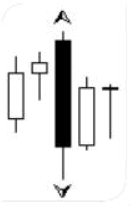# Fractal Analysis on the Forex Market Explained

In this article, we’ll talk about fractals and fractal analysis. How to use fractal analysis? What are the pros and cons of trading fractals in the Forex market?

Before reading the article and writing your questions in the comments section, I recommend to watch this video. It’s not long but covers the biggest part of questions on the topic.

Fractals had been used in trading analysis long before Bill Williams. However, William’s contribution to fractal analysis is invaluable. He did a colossal job finding out that price movements were chaotic.

According to Bill Williams, the market is not a linear but a chaotic system. This is the reason why using linear methods and indicators to predict price action is pretty much useless. The market is a chaotic system, with only temporal periods of consistency.

## FRACTAL ANALYSIS: WHAT MAKES UP A FRACTAL

Forex fractal analysis was developed based on computer modeling. The research found out that the market structure is defined by an inverse correlation.

A fractal is a recurring pattern that can occur on any timeframes. For a pattern to be a fractal, it must consist of at least 5 consecutive bars, with the middle one being the highest (or the lowest).If there is a bearish reversal, a fractal is pointing upwards. A bullish reversal is signaled by an arrow that points downwards. Sometimes, one bar generates two opposite fractals at the same time.In a down fractal, we should be looking at the price low. In an upward-pointing fractal, we’re should be analyzing at the price high.

A fully formed fractal has several characteristics.

## FRACTAL START

A fractal start is a situation when a fractal is closely followed by another fractal pointing in the opposite direction.## FRACTAL SIGNAL

After a fractal start, a fractal signal occurs on the reverse side of the pattern.## FRACTAL STOP

A fractal stop is the furthest point from either of the previous two fractals.By using this method, you can use fewer stop losses and earn bigger profits.

## PROS AND CONS OF FRACTAL ANALYSIS

Like any other trading technique, fractal analysis has both advantages and disadvantages. To effectively use fractals, you need to be able to analyze multiple timeframes and see the big picture of what’s going in the market. The Williams’ method is a trend trading strategy. To identify entry points, you need to use a trend on a high timeframe.

Fractal analysis is used in a variety of Forex strategies and techniques. If used wisely, the fractal analysis will become a great addition to your toolbox, enhancing the profitability of your Forex currency trading.